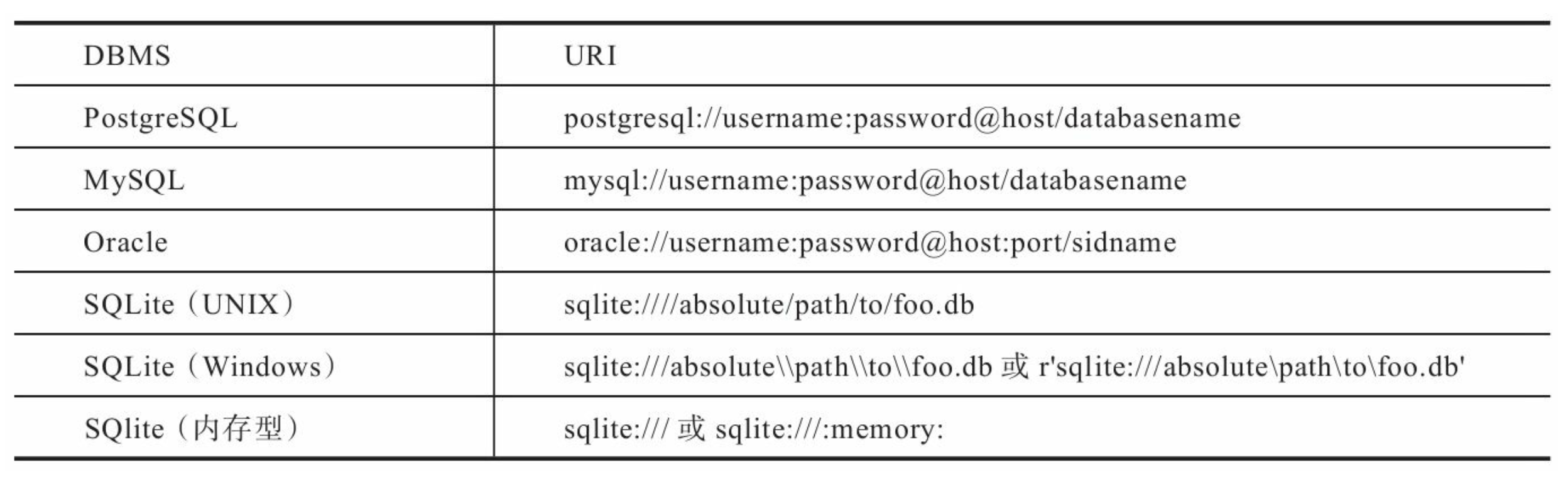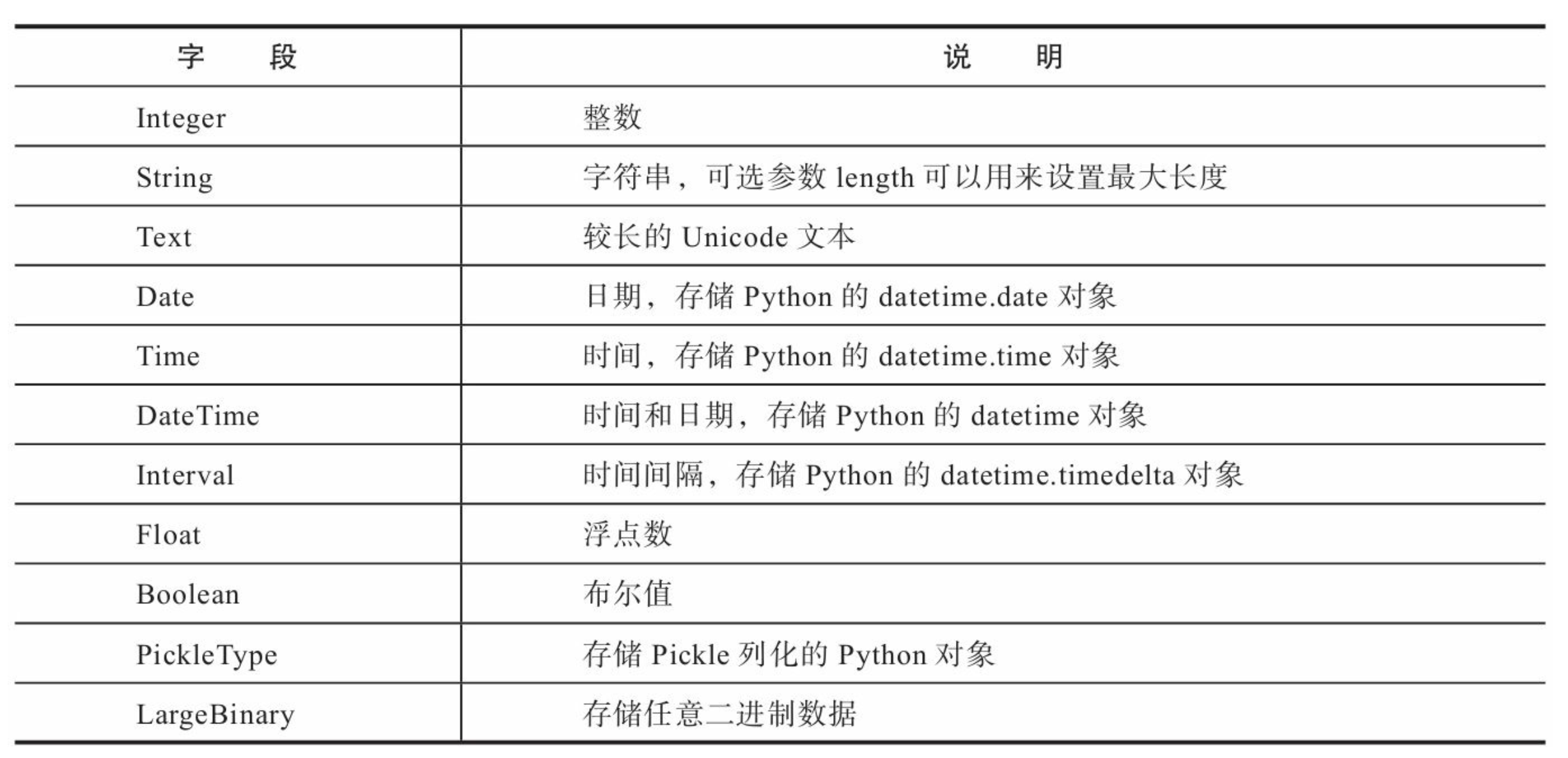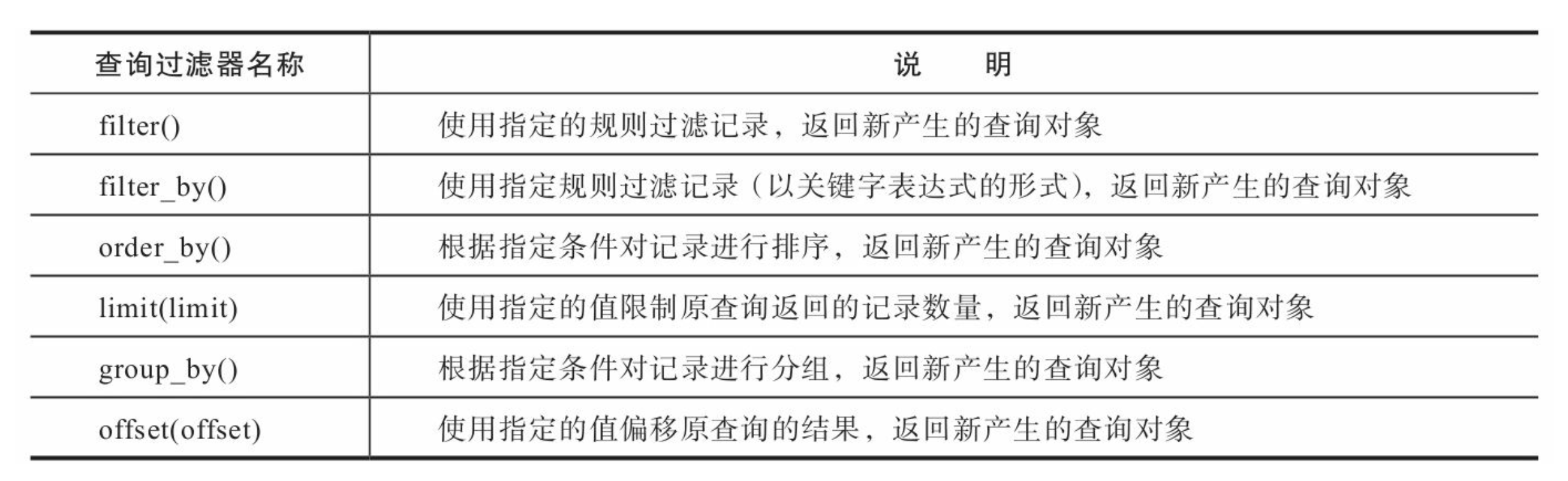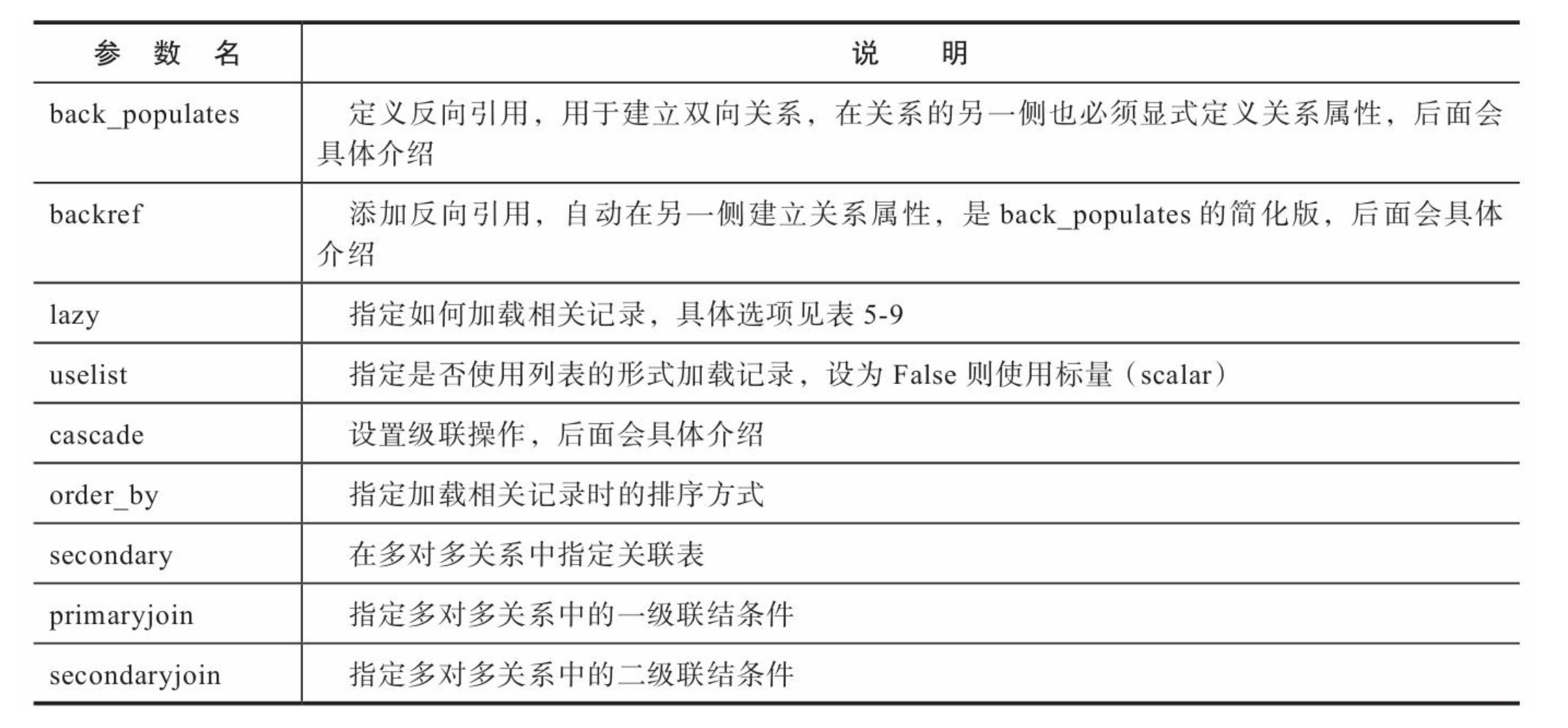### 二、初始配置

#### 1. 安装

> pip install flask-sqlclchemy


#### 2. 扩展的初始化:

from flask import Flask
db = SQLAlchemy(app)


#### 3. 连接数据库服务器<##### 3.1 配置SQLite数据库URI
import os
...
app.config['SQLALCHEMY_DATABASE_URI'] = os.getenv('DATABASE_URL', 'sqlite:///' + os.path.join(app.root_path, 'db.sqlite3'))
app.config['SQLALCHEMY_TRACK_MODIFICATIONS'] = False


##### 3.2 配置Mysql数据库URI
import os
...
app.config['SQLALCHEMY_TRACK_MODIFICATIONS'] = False


app.config['SQLALCHEMY_TRACK_MODIFICATIONS'] = False


### 三、定义数据库模型这里我就演示了下几个常用的

from datetime import datetime
...
class Note(db.Model):
id = db.Column(db.Integer, primary_key=True)
author = db.Column(db.String(25), nullable=False)
created_time = db.Column(db.DateTime, default=datetime.now, nullable = False)
body = db.Column(db.Text,default='Write Something')


Message --> message # 单个单词转换为小写
FooBar --> foo_bar # 多个单词转换为小写并使用下划线分隔

Note类对应的表名称即note。如果你想自己指定表名称，可以通过定义 __tablename__属性来实现。

### 四、创建数据库表

import click
...
@app.cli.command()
@click.option('--drop', is_flag=True, help='Create after drop.')
def initdb(drop):
if drop:
click.confirm('This operation will delete the database, do you want to continue?', abort=True)
db.drop_all()
click.echo('Drop tables.')
db.create_all()
click.echo('Initialized database.')


### 五、数据库操作

# 传入shell上下文
@app.shell_context_processor
def make_shell_context():
return dict(db=db, Note=Note)


> flask initdb --drop
This operation will delete the database, do you want to continue? [y/N]: y
Drop tables.
Initialized database.
Python 3.7.5 (default, Nov 29 2019, 17:17:51)
[Clang 11.0.0 (clang-1100.0.20.17)] on darwin
App: app [production]
>>>


>>> note1 = Note(body='hello')
>>> note2 = Note(body='world')
>>> db.session.commit() # 每次更改都要commit才能同步到数据库
>>> db.session.delete(note1) # 删
>>> db.session.commit()
>>> note1.body = 'giao!' # 改
>>> db.session.commit()
>>> Note.query.filter_by(body='giao!').first() == note1 # 查
TrueNote.query.filter_by(body='SHAVE').first()
# 等价于 Note.query.filter(Note.body='SHAVE').first()


### 六、建立模型之间几种基础的关系模式

#### 1.单向和双向关系

class Writer(db.Model):
id = db.Column(db.Integer, primary_key=True)
name = db.Column(db.String(70), unique=True)
books = db.relationship('Book', back_populates='writer')
# books = db.relationship('Book')
class Book(db.Model):
id = db.Column(db.Integer, primary_key=True)
title = db.Column(db.String(50), index=True)
writer_id = db.Column(db.Integer, db.ForeignKey('writer.id'))
writer = db.relationship('Writer', back_populates='books')
# writer = db.relationship('Writer')


relationship()函数的第一个参数为关系另一侧的模型名称，在Writer中它会告诉 SQLAlchemy将Writer类与Book类建立关系【下面几种关系都默认使用双向关系】

#### 2.一对多/多对一

class Book(db.Model):
...
writer_id = db.Column(db.Integer, db.ForeignKey('writer.id'))


class Writer(db.Model):
id = db.Column(db.Integer, primary_key=True)
name = db.Column(db.String(70), unique=True)
books = db.relationship('Book', back_populates='writer')

class Book(db.Model):
id = db.Column(db.Integer, primary_key=True)
title = db.Column(db.String(50), index=True)
writer_id = db.Column(db.Integer, db.ForeignKey('writer.id'))
writer = db.relationship('Writer', back_populates='books')


#### 3.一对一

class Writer(db.Model):
id = db.Column(db.Integer, primary_key=True)
name = db.Column(db.String(70), unique=True)
book = db.relationship('Book', back_populates='writer', uselist=False)

class Book(db.Model):
id = db.Column(db.Integer, primary_key=True)
title = db.Column(db.String(50), index=True)
writer_id = db.Column(db.Integer, db.ForeignKey('writer.id'))
writer = db.relationship('Writer', back_populates='books')


#### 4.多对多

association_table = db.Table('association',db.Column('student_id', db.Integer, db.ForeignKey('student.id')),db.Column('teacher_id', db.Integer, db.ForeignKey('teacher.id'))
)
class Student(db.Model):
id = db.Column(db.Integer, primary_key=True)
name = db.Column(db.String(70), unique=True)
teachers = db.relationship('Teacher',
secondary=association_table, back_populates='students')
class Teacher(db.Model):
id = db.Column(db.Integer, primary_key=True)
name = db.Column(db.String(70), unique=True)
office = db.Column(db.String(20))### 七、迁移数据库

#### 1.安装

> pip install flask-migrate


#### 2.实例化

from flask import Flask
...
db = SQLAlchemy(app)
migrate = Migrate(app, db) # 在db对象创建后调用


#### 3.创建迁移环境

> flask db init


#### 4.生成迁移脚本

> flask db migrate -m "add note timestamp"
...
INFO [alembic.autogenerate.compare] Detected added column 'message.timestamp


-m 选项用来添加迁移备注信息。

【迁移命令是由Alembic自动生成的，其中可能包含错误，所以有必要在生成后检查一下。】

#### 5.更新数据库

> flask db upgrade